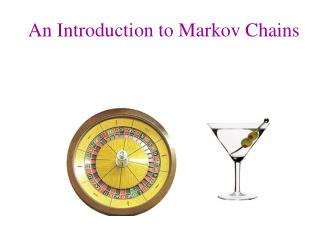# An Introduction to Markov Chains - PowerPoint PPT PresentationDownload PresentationAn Introduction to Markov Chains

An Introduction to Markov Chains
Download Presentation## An Introduction to Markov Chains

- - - - - - - - - - - - - - - - - - - - - - - - - - - E N D - - - - - - - - - - - - - - - - - - - - - - - - - - -
##### Presentation Transcript

1. An Introduction to Markov Chains

2. Homer and Marge repeatedly play a gambling game. Each time they play,the probability that Homer wins is 0.4, and the probability that Homer loses is 0.6 A “Drunkard’s Walk”

3. 0 1 2 3 4 0 1 2 3 4 P(Homer wins) = .4 P(Homer loses) = .6 Homer and Marge both start with \$2

4. A Markov Chain is a mathematical model for a process which moves step by step through various states. In a Markov chain, the probability that the process moves from any given state to any other particular state is always the same, regardless of the history of the process.

5. A Markov chain consists of states and transitionprobabilities. Each transition probability is the probability of moving from one state to another in one step. The transition probabilities are independent of the past, and depend only on the two states involved. The matrix of transition probabilities is called the transition matrix.

6. 0 1 2 3 4 P(Homer wins) = .4 P(Homer loses) = .6 Homer and Marge both start with \$2

7. If Pis the transition matrix for a Markov Chain, then the nth power of P gives the probabilities of going from state to state in exactly n steps.

8. If the vector v represents the initial state, then the probabilities of winding up in the various states in exactly n steps are exactly v times the nth power of P .

9. When they both start with \$2, the probability that Homer is ruined is 9/13. If Homer starts with \$ x and Marge starts with \$ N-x, and P(Homer wins) = p, P(Homer loses) = q, then the probability Homer is ruined is

10. Suppose you be on red in roulette. P(win) = 18/38 = 9/19; P(lose) = 10/19. Suppose you and the house each have \$10 Now suppose you have \$ 10 and the house has \$20

11. Now suppose you and the house each have \$100.

12. Andrei Markov (1856-1922) Paul Eherenfest: Diffusion model, early 1900sStatistical interpretation of the second law of thermodynamics: The entropy of a closed system can only increase.Proposed the “Urn Model” to explain diffusion. Albert Einstein, 1905Realized Brownian motion would provide a magnifying glass into the world of the atom. Brownian motion has been extensively modeled by Markov Chains

13. Particles are separated by a semi-permeable membrane, which they can pass through in either direction. Suppose that there are N black particles inside the membrane, and N white particles outside the membrane. Each second, one random molecule goes from outside the membrane to inside, and vice versa. There are N+1 states, given by the number of white molecules inside. Osmosis

14. 0 1 2 3 4 5 5 molecules

15. N molecules

16. N molecules

17. If this process runs for a while, an interesting question is: How much time, on average, is the process in each state? A Markov chain with transition matrix P is said to be regular if some power of P has all positive entries for some n. In a regular Markov chain, it is possible to get from any state to any other state in n steps.

18. The Markov chain for our osmosis process is regular. Even starting with all black particles inside, if a white particle entered at every step, then the process would pass from zero white inside through all possible states.

19. For a regular Markov chain, the amount of time the process spends in each state is given by the fixed probability vector, which is the vector a such that Pa = a. Moreover, for any probability vector w, No matter what the starting state, if the process runs for a long time, the probability of being in a given state is given by a.

20. In the long run, the fraction of time the process spends in each state is given by the fixed probability vector.

21. For N particles, the fixed vector is: 1 1 1 1 2 1 1 3 3 1 1 4 6 4 1 1 5 10 10 5 1 1 6 15 20 15 6 1

22. Fixed vectors N = 4 (1/70, 16/70, 36/70, 16/70, 1/70)

23. Now suppose 500 molecules The percent of the time that there are between 225 and 275 black molecules inside is 0.999. The percent of the time that there are either fewer than 100 black or more than 400 black molecules inside is

24. If the universe is 15 billion years old, the average amount of time that a system with 500 molecules will have fewer than 100 black or more than 400 black molecules inside the membrane is